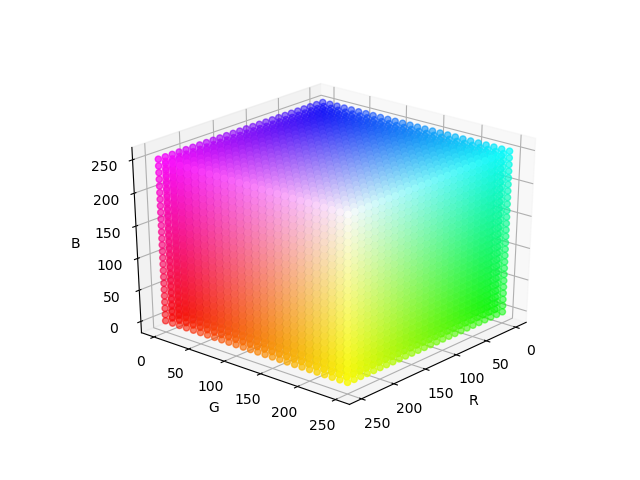# Color models

Institute for Environmental and Spatial Analysis...University of North Georgia

## 1   Color model

A color model (also referred to as color space or color model) provides a standard specification of colors and defines a coordinate system.

Each color is represented by a single point within a subspace of this coordinate system.

• RGB (red, green, blue) model for color displays
• CMY (cyan, magenta, yellow) and CMYK (CMY, black) models for color printing
• HSI (hue, saturation, intensity) model for decoupling the color and intensity information (e.g., human interpretation)

## 2   RGB color model

• Based on a Cartesian coordinate system
• Consists of the primaries of light (mainly for displays)
• Red, green, and blue axes
• RGB color cube normalized to $[0,1]^3$
• Red, green, and blue at three corners on the axes
• Cyan, magenta, and yellow at the other three corners
• Grayscale (equal RGB values) extends from black to white
• $k$ bits per primary or total $3k$ bits $\rightarrow\left(2^k\right)^3=2^{3k}\text{ colors}$
• 24-bit (8 bits per primary) image $\rightarrow 2^{24}=16{,}777{,}216\text{ colors}$
• 24-bit color images are often referred to as full-color images

### 2.1   RGB color cubergb_color_cube.py

### 2.2   RGB color planes

rgb_color_planes.py

### 2.3   RGB color componentsrgb_color_components.py

### 2.4   Safe RGB colors

Not all color display systems can produce full-color images. Safe RGB colors are “a subset of colors that are likely to be reproduced faithfully, reasonably independently of viewer hardware capabilities” (Gonzalez and Woods, 2002). Also called:

• All-systems-safe colors
• Safe Web colors
• Safe browser colors

Based on the assumption that 256 is the minimum number of colors that can faithfully be reproduced by any system, it would be useful to define a set of standard colors. However, it is known that different operating systems produce 40 of these 256 colors differently, so the remaining 216 colors have become the safe RGB colors.

These colors can be reproduced by RGB triplets of 6 values (0, 51, 102, 153, 204, or 255). Note that the intensity increment is 51, all values are divisible by 3, and the number of colors is $6^3=216$.

### 2.5   Hexadecimal representation of safe RGB colors

Each primary in safe RGB colors ranges from 0 to 255, which is equivalent to 0 to FF in hexadecimal notation.

Padding the hexadecimal number for each primary with 0 when it is less than $(10)_{16}$ and concatenating three hexadecimal numbers produce the hexadecimal representation of a safe RGB color.

For example, 000000 and FFFFFF represent black and white, respectively.

What is red?

FF0000

Can you make a gray?

## 3   CMY color model

### 3.1   RGB-to-CMY conversion

\begin{align*} \begin{bmatrix} C\\ M\\ Y \end{bmatrix}& =\begin{bmatrix} 1\\ 1\\ 1 \end{bmatrix}-\begin{bmatrix} R\\ G\\ B \end{bmatrix}\\ \begin{bmatrix} R\\ G\\ B \end{bmatrix}& =\begin{bmatrix} 1\\ 1\\ 1 \end{bmatrix}-\begin{bmatrix} C\\ M\\ Y \end{bmatrix} \end{align*}

RGB and CMY are normalized to $[0,1]^3$.

### 3.2   Exercise: RGB-to-CMY conversion

1. 70% R, 20% G, 10% B $\rightarrow$ CMY %?
2. In a 24-bit image, R=125, G=90, B=230 $\rightarrow$ CMY on a 24-bit printed medium?
3. 30% C, 15% M, 55% Y $\rightarrow$ RGB %?
4. 35% C, 7% M, 58% Y $\rightarrow$ RGB on a 21-bit color display?
5. In a 24-bit printed image, C=225, M=105, Y=35 $\rightarrow$ RGB on a 24-bit color display?

## 4   HSI color model

The HSI color model is created by slicing the RGB color cube perpendicular to the grayscale line (the intensity axis in HSI).

That is, the HSI color model decouples the intensity from RGB and creates HS planes.

### 4.1   RGB color cube slicing### 4.2   HS plane### 4.3   RGB-to-HSI conversion

\begin{align*} I&=\frac{R+G+B}{3}\\ H&=\begin{cases} \theta&\text{ if }G\geq B\\ 360^\circ-\theta&\text{ otherwise} \end{cases}\\ \theta&=\cos^{-1}\left\{\frac{\frac{1}{2}\left[(R-G)+(R-B)\right]}{\left[(R-G)^2+(R-B)(G-B)\right]^{1/2}}\right\}\\ S&=1-\frac{\min(R,G,B)}{I} \end{align*}

RGB is normalized to $[0,1]^3$.

We can normalize H to $[0,1]$ by dividing it by 360° so that HSI is in $[0,1]^3$. S and I are already within $[0,1]$.

### 4.4   HSI-to-RGB conversion

For $0^\circ\leq H<120^\circ$, \begin{align*} B&=I(1-S)\\ R&=I\left[1+\frac{S\cos H}{\cos(60^\circ-H)}\right]\\ G&=3I-(R+B) \end{align*}

For $240^\circ\leq H<360^\circ$, \begin{align*} H&=H-240^\circ\\ G&=I(1-S)\\ B&=I\left[1+\frac{S\cos H}{\cos(60^\circ-H)}\right]\\ R&=3I-(G+B) \end{align*}

For $120^\circ\leq H<240^\circ$, \begin{align*} H&=H-120^\circ\\ R&=I(1-S)\\ G&=I\left[1+\frac{S\cos H}{\cos(60^\circ-H)}\right]\\ B&=3I-(R+G) \end{align*}

The normalized $H$ needs to be multiplied by 360° first.

If $H$ is not normalized, it is already in degrees and should not be multiplied by 360°.

### 4.5   Maximum S

S is the ratio of the Euclidean distance of a color from the intensity axis to that of the fully saturated color in the same hue. For example, colors on the R-G, G-B, and B-R surfaces all have S=1 regardless of their Euclidean distance from the intensity axis. For $I>\frac{2}{3}$, no colors are on these surfaces and the maximum S is smaller than 1. We can derive the maximum S, $S_\text{max}$, given H and I by restricting the R, G, and B equations within $[0,1]$.

For $0^\circ\leq H<30^\circ$, $S_\text{max}=\min\left[1, \left(\frac{1}{I}-1\right)\frac{\cos(60^\circ-H)}{\cos H}, -\frac{\cos(60^\circ-H)}{\cos(60^\circ-H)-\cos H}\right]$

For $30^\circ\leq H<90^\circ$, $S_\text{max}=\min\left[1, \left(\frac{1}{I}-1\right)\frac{\cos(60^\circ-H)}{\cos H}, \left(\frac{1}{I}-1\right)\frac{\cos(60^\circ-H)}{\cos(60^\circ-H)-\cos H}\right]$

For $90^\circ\leq H<120^\circ$, $S_\text{max}=\min\left[1, -\frac{\cos(60^\circ-H)}{\cos H}, \left(\frac{1}{I}-1\right)\frac{\cos(60^\circ-H)}{\cos(60^\circ-H)-\cos H}\right]$

H is the final H for the R, G, and B equations. For example, for $120^\circ\leq H<240^\circ$, $H=H-120^\circ$.

### 4.6   Exercise: RGB-to-HSI conversion

1. 70% R, 20% G, 10% B $\rightarrow$ HSI with the non-normalized H?
2. 60% R, 10% G, 30% B $\rightarrow$ HSI with the normalized H?
3. In a 24-bit image, R=125, G=90, B=230 $\rightarrow$ HSI with the normalized H?

### 4.7   Exercise: HSI-to-RGB conversion

1. H=120°, S=0.2, I=0.7 $\rightarrow$ normalized RGB?
2. H=0.4, S=0.9, I=0.3 $\rightarrow$ RGB on a 24-bit color display?
3. H=0.6, S=0.1, I=0.5 $\rightarrow$ CMY on a 24-bit printed medium?
4. H=0, S=1, I=1/3 $\rightarrow$ Color name?
5. H undefined, S undefined, I=0 $\rightarrow$ Color name?
6. H undefined, S=0, I=1 $\rightarrow$ Color name?
7. H undefined, S undefined or S=0 $\rightarrow$ Grayscale

## 5   Homework: Color model conversions

1. Convert C=63, M=35, Y=10 on a 18-bit printed medium to HSI with the normalized H.
2. Convert H=0.1, S=0.2, and I=0.8 to CMY on a 18-bit printed medium.